# Selva Prabhakaran

Selva is the Chief Author and Editor of Machine Learning Plus, with 4 Million+ readership. He has authored courses and books with100K+ students, and is the Principal Data Scientist of a global firm.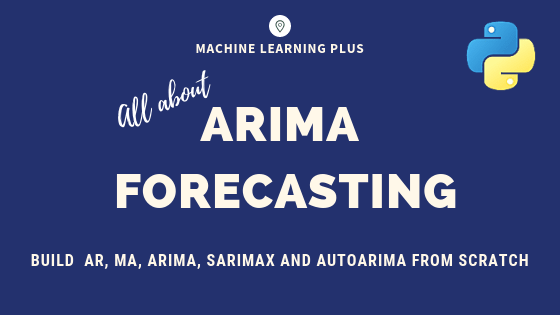## ARIMA Model – Complete Guide to Time Series Forecasting in Python

Using ARIMA model, you can forecast a time series using the series past values. In this post, we build an optimal ARIMA model from scratch and extend it to Seasonal ARIMA (SARIMA) and SARIMAX models. You will also see how to build autoarima models in python Master complete Time Series Concepts and Implementation with my …

## One Sample T Test – Clearly Explained with Examples | ML+

One sample T-Test tests if the given sample of observations could have been generated from a population with a specified mean. If it is found from the test that the means are statistically different, we infer that the sample is unlikely to have come from the population. For example: If you want to test a …

## Understanding Standard Error – A practical guide with examples

Standard error of the mean measures how spread out the means of the sample can be from the actual population mean. Standard error allows you to build a relationship between a sample statistic (computed from a smaller sample of the population) and the population’s actual parameter. Standard Error – A practical guide with examples. Photo …

## Confidence Interval – Fully Explained

Confidence interval is a measure to quantify the uncertainty in an estimated statistic (like the mean) when the true population parameter is unknown. Training Custom Text Classification Model in spaCy. Photo by Jessica Wong. You will know 1. What is Confidence Interval? 2. Two types of Confidence Intervals problems 3. Difference between Population parameter vs …

## T Test (Students T Test) – Understanding the math and how it works

T Test (Students T Test) is a statistical significance test that is used to compare the means of two groups and determine if the difference in means is statistically significant. In this one, you’ll understand when to use the T-Test, the different types of T-Test, math behind it, how to determine which test to choose …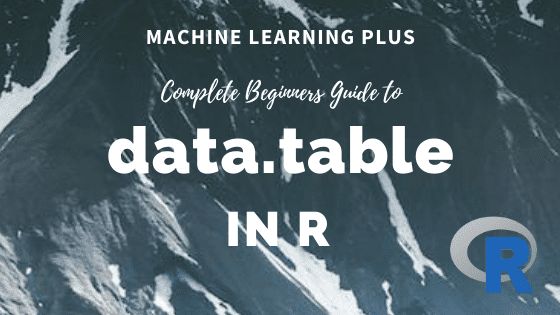## data.table in R – The Complete Beginners Guide

data.table is a package is used for working with tabular data in R. It provides the efficient data.table object which is a much improved version of the default data.frame. It is super fast and has intuitive and terse syntax. If you know R language and haven’t picked up the data.table package yet, then this tutorial …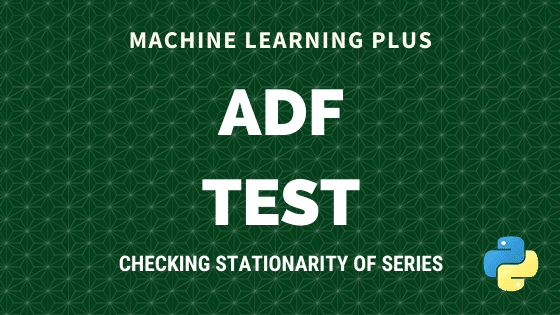Augmented Dickey Fuller test (ADF Test) is a common statistical test used to test whether a given Time series is stationary or not. It is one of the most commonly used statistical test when it comes to analyzing the stationary of a series. 1. Introduction In ARIMA time series forecasting, the first step is to …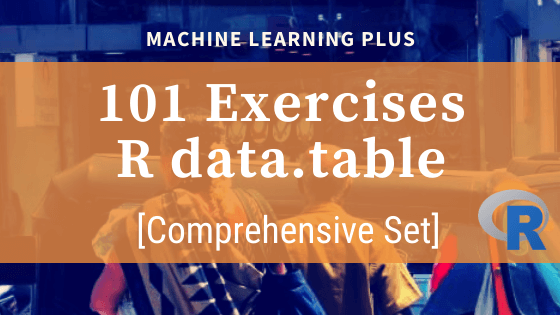## 101 R data.table Exercises

The data.table package in R is super fast when it comes to handling data. It has a syntax that reduces keystrokes while making R code easier to read. These set of exercises are designed to help you to oil your data brain through solving data manipulation exercises. Related post: 101 Python datatable Exercises (pydatatable) 101 …## What is P-Value? – Understanding the meaning, math and methods

P Value is a probability score that is used in statistical tests to establish the statistical significance of an observed effect. Though p-values are commonly used, the definition and meaning is often not very clear even to experienced Statisticians and Data Scientists. In this post I will attempt to explain the intuition behind p-value as …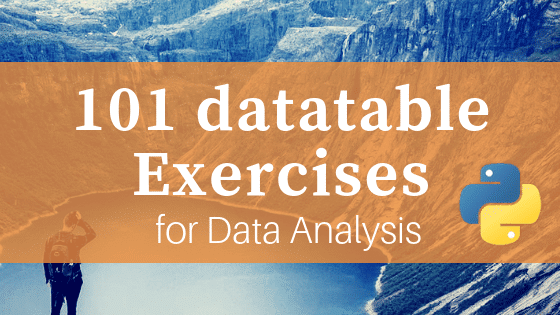## 101 Python datatable Exercises (pydatatable)

Python datatable is the newest package for data manipulation and analysis in Python. It carries the spirit of R’s data.table with similar syntax. It is super fast, much faster than pandas and has the ability to work with out-of-memory data. Looking at the performance it is on path to become a must-use package for data …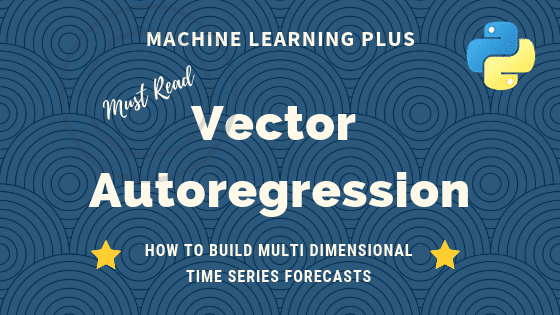## Vector Autoregression (VAR) – Comprehensive Guide with Examples in Python

Vector Autoregression (VAR) is a forecasting algorithm that can be used when two or more time series influence each other. That is, the relationship between the time series involved is bi-directional. In this post, we will see the concepts, intuition behind VAR models and see a comprehensive and correct method to train and forecast VAR …## Mahalanobis Distance – Understanding the math with examples (python)

Mahalanobis distance is an effective multivariate distance metric that measures the distance between a point and a distribution. It is an extremely useful metric having, excellent applications in multivariate anomaly detection, classification on highly imbalanced datasets and one-class classification. This post explains the intuition and the math with practical examples on three machine learning use …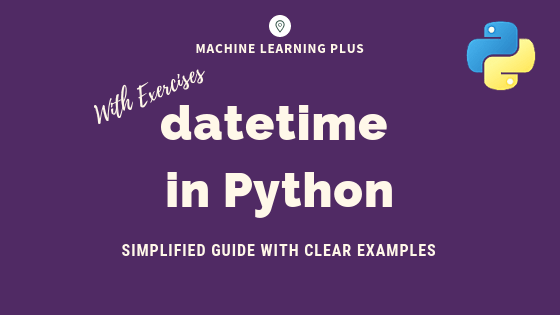## datetime in Python – Simplified Guide with Clear Examples

datetime is the standard module for working with dates in python. It provides 4 main objects for date and time operations: datetime, date, time and timedelta. In this post you will learn how to do all sorts of operations with these objects and solve date-time related practice problems (easy to hard) in Python. datetime in …## Principal Component Analysis (PCA) – Better Explained

Principal Components Analysis (PCA) is an algorithm to transform the columns of a dataset into a new set of features called Principal Components. By doing this, a large chunk of the information across the full dataset is effectively compressed in fewer feature columns. This enables dimensionality reduction and ability to visualize the separation of classes …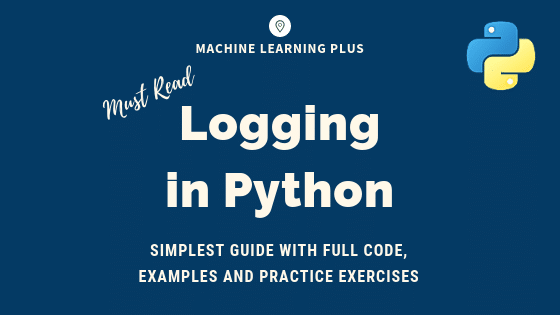## Python Logging – Simplest Guide with Full Code and Examples

The logging module lets you track events when your code runs so that when the code crashes you can check the logs and identify what caused it. Log messages have a built-in hierarchy – starting from debugging, informational, warnings, error and critical messages. You can include traceback information as well. It is designed for small …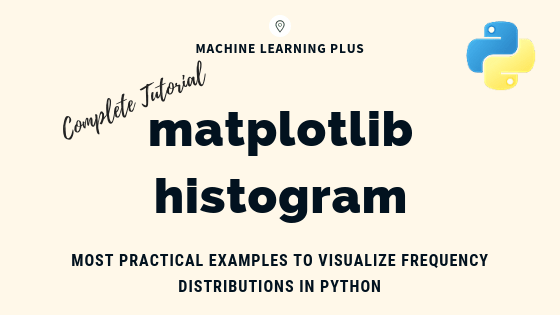## Matplotlib Histogram – How to Visualize Distributions in Python

Matplotlib histogram is used to visualize the frequency distribution of numeric array by splitting it to small equal-sized bins. In this article, we explore practical techniques that are extremely useful in your initial data analysis and plotting.   Create Powerful Visualizations using Python with my FREE 9-Day-Video-Course.    Content What is a histogram? How to …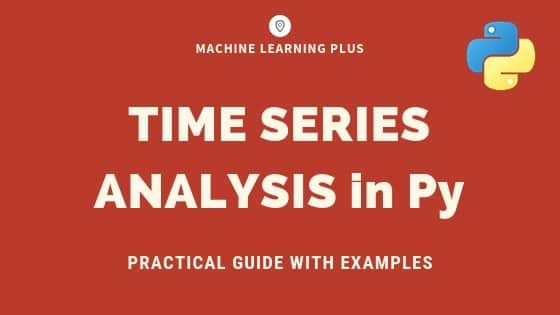## Time Series Analysis in Python – A Comprehensive Guide with Examples

Time series is a sequence of observations recorded at regular time intervals. This guide walks you through the process of analyzing the characteristics of a given time series in python.   Time Series Analysis in Python – A Comprehensive Guide. Photo by Daniel Ferrandiz. Contents What is a Time Series? How to import Time Series …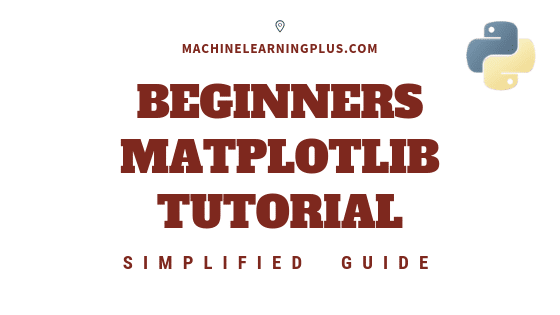## Matplotlib Tutorial – A Complete Guide to Python Plot with Examples

This tutorial explains matplotlib’s way of making plots in simplified parts so you gain the knowledge and a clear understanding of how to build and modify full featured matplotlib plots. 1. Introduction Matplotlib is the most popular plotting library in python. Using matplotlib, you can create pretty much any type of plot. However, as your …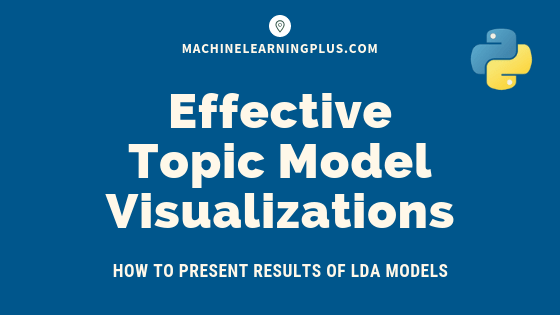## Topic modeling visualization – How to present the results of LDA models?

In this post, we discuss techniques to visualize the output and results from topic model (LDA) based on the gensim package. Become a high paid data scientist with my structured Machine Learning Career Path. Includes access to all my current and future courses of Machine Learning, Deep Learning and Industry Projects. With 24×7 query support. …Course Preview

## Machine Learning A-Z™: Hands-On Python & R In Data Science

### Free Sample Videos:#### Machine Learning A-Z™: Hands-On Python & R In Data Science#### Machine Learning A-Z™: Hands-On Python & R In Data Science#### Machine Learning A-Z™: Hands-On Python & R In Data Science#### Machine Learning A-Z™: Hands-On Python & R In Data Science